Methods and formulas for Predict for Binary Logistic

Find the method or formula of your choice.

Fitted and predicted values

To calculate the prediction, invert the link function for the model, which is the logit link function. The formula is:Notation

TermDescription
exp(·) the exponential function
X' the transpose of the vector of points to predict forthe vector of estimated coefficients

Standard error of fitted values and predictions

Notation

TermDescription
Φ1, for the binomial and Poisson models
xhthe vector of a new design point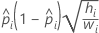the transpose of xh
Xthe design matrix
Wthe weight matrix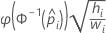the first derivative of the link function evaluated at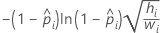the predicted mean response

Confidence limits for fits and predictions

The confidence limits use the Wald approximation method. This is the formula for a 100(1 − α)% two-sided confidence interval:

Notation

TermDescription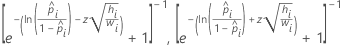the inverse of the link function evaluated at x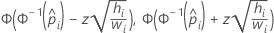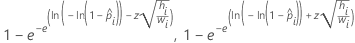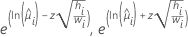the transpose of the vector of the predictors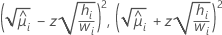the vector of estimated coefficients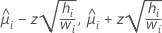the value of the inverse cumulative distribution function for the normal distribution evaluated at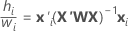αthe significance level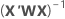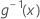Xthe design matrix
Wthe weight matrix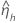1, for binomial and Poisson models
By using this site you agree to the use of cookies for analytics and personalized content.  Read our policy